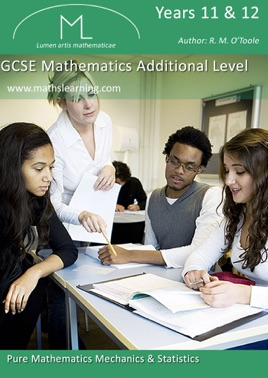• 3,99 €

## Description de l’éditeur

Description:

The GCSE Additional Maths specification is designed for those students who require a knowledge of mathematics beyond GCSE Higher Tier Maths and who are capable of working beyond the limits of the GCSE maths specification.

Knowledge of GCSE Higher Tier Mathematics will be assumed.

Our GCSE iBooks 1, 2 and 3 and our iBook2 GCSE Revision Test are designed to be used by students of mathematics up to GCSE Higher Tier.

The scheme of examination is a broad-based scheme of assessment in which candidates are required to take two written papers. Paper 1 (Pure) and Paper 2 (Mechanics along with Statistics or ICT).

Keywords

Pure Maths:

Matrices, Transformation Matrices, Indices and Logarithms

Mechanics:
Vectors, Constant Acceleration, Calculus, Differentiation, Integration, Displacement, Velocity, Motion, Newton's Laws, Forces, Resolving Forces

Statistics:
Ogive, Interquartile Range, Mean, Median, Mode, Frequency, Histogram, Frequency Polygon,
Frequency Density, Cumulative Frequency,
Box and Whisker diagram, Stem and Leaf diagram,

Scatter diagram, Correlation, Probability,
Conditional Probability, Tree Diagram, And/Or Rules